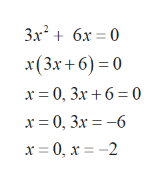# Find the coordinates of the x-intercepts of the graph of the equation. (If an answer does not exist, enter DNE.)y = 3x2 + 6x(x, y) =     (smaller x-value)(x, y) =     (larger x-value)

Question
1 views

Find the coordinates of the x-intercepts of the graph of the equation. (If an answer does not exist, enter DNE.)

y = 3x2 + 6x
(x, y) =

(smaller x-value)
(x, y) =

(larger x-value)
check_circle

Step 1

Given, the graph of the equation

Step 2

To find the coordinates of x-intercepts.

Set y=0 to find x-intercept. Thenhelp_outlineImage Transcriptionclose3x? + 6х — 0 x(3х +6) - 0 х3D0, 3х + 6 3 0 х3D0, 3х — —6 х 3D0, х 3 -2 fullscreen
Step 3

Therefore, the x-intercepts ...

### Want to see the full answer?

See Solution

#### Want to see this answer and more?

Solutions are written by subject experts who are available 24/7. Questions are typically answered within 1 hour.*

See Solution
*Response times may vary by subject and question.
Tagged in

### Polynomials